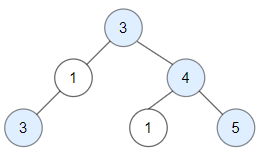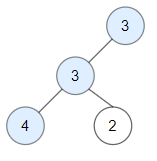# Count Good Nodes in Binary Tree LeetCode Solution

Frequently asked in Amazon Bloomberg Microsoft Salesforce
DRWViews 101

## Problem Statement:

Count Good Nodes in Binary Tree LeetCode Solution:

Given a binary tree `root`, a node X in the tree is named good if in the path from the root to X there are no nodes with a value greater than X.

Return the number of good nodes in the binary tree.

Example 1:Input:

``` root = [3,1,4,3,null,1,5]
```

Output:

``` 4
```

Explanation: Nodes in blue are good. Root Node (3) is always a good node. Node 4 -> (3,4) is the maximum value in the path starting from the root. Node 5 -> (3,4,5) is the maximum value in the path Node 3 -> (3,1,3) is the maximum value in the path.

Example 2:Input:

``` root = [3,3,null,4,2]
```

Output:

``` 3
```

Explanation:  Node 2 -> (3, 3, 2) is not good, because “3” is higher than it.

Example 3:

Input:

``` root = 
```

Output:

``` 1
```

Explanation: Root is considered as good.

Constraints:

• The number of nodes in the binary tree is in the range `[1, 10^5]`.
• Each node’s value is between `[-10^4, 10^4]`.

## Approach:

### Idea:

1. This problem can be solved using the iterative way and also the recursive way. Let’s look into the recursive way as it is easy.
2. we will traverse the right subtree and left subtree recursive manner.
3. Update the maximum value found while recursing down to the paths from the root to the leaves.
4. If node value >= current maximum, we will increase our cnt value.
5. When the node will be null we will simply end our recursion call.
6. Total cnt will be our estimated answer.

## Code:

### Count Good Nodes in Binary Tree C++ Code:

```class Solution {
public:
void Count(TreeNode* root,int &count,int maxi){
if(root==NULL) return;
if(root->val >= maxi){count++; maxi=max(maxi,root->val);}
Count(root->left,count,maxi);
Count(root->right,count,maxi);
return;

}
int goodNodes(TreeNode* root){
int count=0;
int maxi=root->val;
Count(root,count,maxi);
return count;
}
};```

### Count Good Nodes in Binary Tree Java Code:

```class Solution {
int cnt=0;
void rec(TreeNode root,int val)
{

if(root==null)
return ;
if(root.val>=val)
cnt++;
int k=Math.max(val,root.val);
rec(root.left,k);
rec(root.right,k);
}
public int goodNodes(TreeNode root) {
int val=Integer.MIN_VALUE;
rec(root,val);
return cnt;
}
}

```

## Complexity Analysis of Count Good Nodes in Binary Tree Leetcode Solution:

### Time Complexity:

Time complexity will be O(n). Where n is the number of nodes, we are visiting every node only once.

### Space Complexity:

We are not taking any extra space. So, space complexity will be O(1).

Translate »